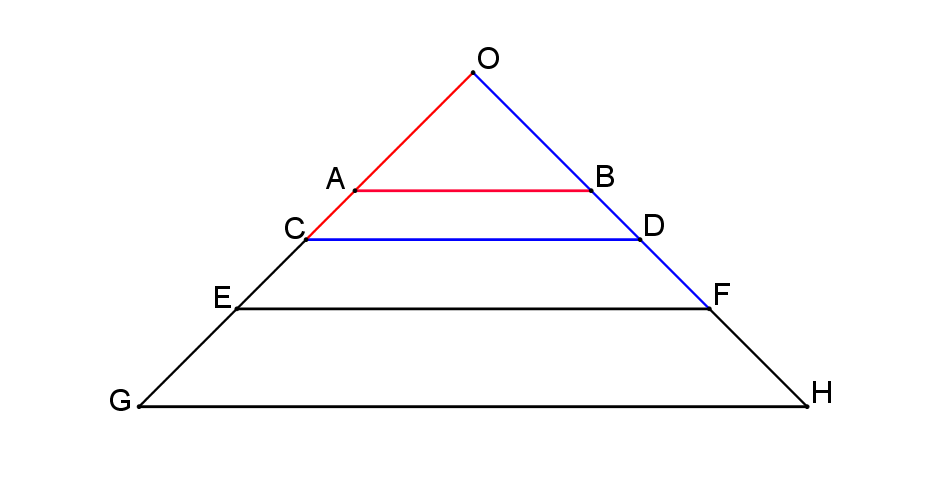#### You may also like### At a Glance

The area of a regular pentagon looks about twice as a big as the pentangle star drawn within it. Is it?### Six Discs

Six circular discs are packed in different-shaped boxes so that the discs touch their neighbours and the sides of the box. Can you put the boxes in order according to the areas of their bases?### Equilateral Areas

ABC and DEF are equilateral triangles of side 3 and 4 respectively. Construct an equilateral triangle whose area is the sum of the area of ABC and DEF.

# Towering Trapeziums

### Why do this problem?

This problem gives students an opportunity to do some geometrical reasoning and apply what they know about Pythagoras' theorem, area of triangles, and sequences.

### Possible approach

Show the image:"This diagram was created by starting with a right angled isosceles triangle $OAB$ with area 1 unit."
"$AB$, $CD$, $EF$, and $GH$ are all parallel."
"$OC$ and $AB$ have the same length."
"$OE$ and $CD$ have the same length."
"$OG$ and $EF$ have the same length."

Invite students to construct the diagram for themselves, and then pose the following questions:
"Can you find the area of the trapezium $ABDC?$"
"What about trapezium $CDFE?$"
"What about trapezium $EFHG?$"

Give students some time to explore the problem, at first on their own and then with a partner. As they are working, circulate and listen out for useful strategies to discuss in a mini-plenary. If no useful strategies emerge, share with students the advice offered in Getting Started.

Any students who work out the areas quickly can consider the following: "If the pattern continued, what would be the area of the $n^{th}$ trapezium in the chain?"

Finish by discussing what students found and what strategies they used to work out the relevant areas.

### Key questions

Can you find an expression for the area of an isosceles right-angled triangle with two sides of length $a$?
Can you find an expression for the area of an isosceles right-angled triangle with hypotenuse $b$?

### Possible extension

Another challenge involving areas and Pythagoras' Theorem is Compare Areas.

### Possible support

Some students may benefit from working on some of the Pythagoras' Theorem Short Problems before looking at this problem.